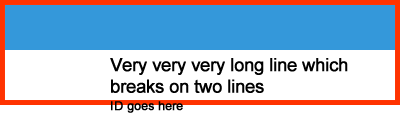# Stretching a RelativeLayout's height with an expandable StackLayout

Hi,

Here is my code:

```<RelativeLayout>
<BoxView Color="#3498DA" HeightRequest="50" RelativeLayout.WidthConstraint="{ConstraintExpression Type=RelativeToParent, Property=Width, Factor=1}"/>

<StackLayout
RelativeLayout.XConstraint="{ConstraintExpression Type=RelativeToParent, Factor=0, Property=X, Constant=108}"
RelativeLayout.YConstraint="{ConstraintExpression Type=RelativeToParent, Factor=0, Property=Y, Constant=53}"
RelativeLayout.WidthConstraint="{ConstraintExpression Type=RelativeToParent, Factor=1, Property=Width, Constant=-118}"
VerticalOptions="StartAndExpand">

<Label Text="{Binding Name}" FontSize="18" FontAttributes="Bold"/>
<Label Text="{Binding Id, StringFormat='ID: {0}'}" FontSize="Micro"/>
</StackLayout>
</RelativeLayout>
```

Note: I used a StackLayout in order to get the ID label systematically following the Name label.

This works fine when Name label is shown on 1 line:But whenever the name is shown on 2 lines, then basically the RelativeLayout is not stretched enough (red borders show how Xamarin computes the height of the RelativeLayout:Question: How should I handle this properly?

Remark: Using a RelativeLayout because on the left of the labels there is some graphics, similarly to https://developer.xamarin.com/samples/xamarin-forms/UserInterface/BusinessTumble/

Tagged: